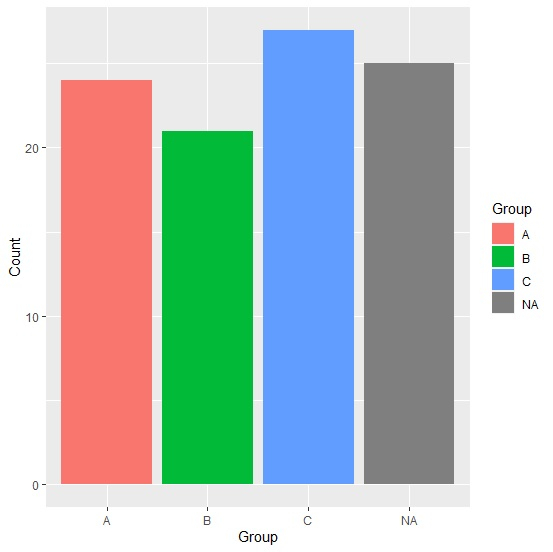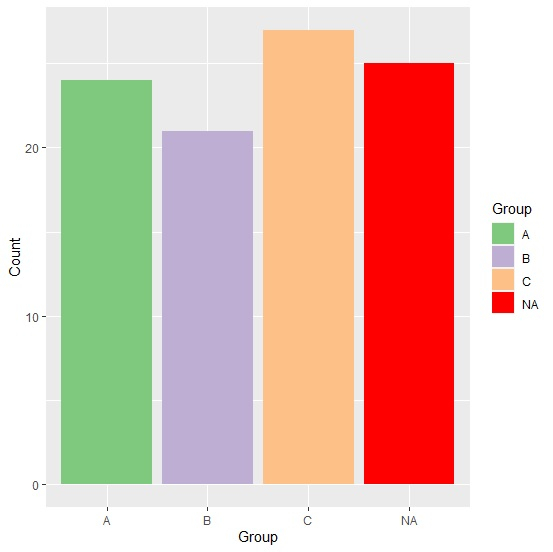# How to display NA frequency for a ggplot2 graph using color brewer in R?

To display NA frequency for a ggplot2 graph using color brewer in R, we can follow the below steps −

• First of all, create a data frame.
• Then, create the chart with default colors.
• After that, use scale_colour_brewer function to create the bar chart and set the color of NA values bar with na.value.

## Create the data frame

Let's create a data frame as shown below −

Live Demo

Group<-c("A","B","C",NA)
Count<-c(24,21,27,25)
df<-data.frame(Group,Count)
df

On executing, the above script generates the below output(this output will vary on your system due to randomization) −

   Group Count
1   A    24
2   B    21
3   C    27
4 <NA>   25

## Create the bar chart with default colors

Loading ggplot2 package and creating the bar for the data in df −

Group<-c("A","B","C",NA)
Count<-c(24,21,27,25)
df<-data.frame(Group,Count)
library(ggplot2)
ggplot(df,aes(Group,Count,fill=Group))+geom_bar(stat="identity")

#### Output## Create the bar chart with color brewer and change the color of bar representing NA

Use scale_colour_brewer function of ggplot2 package to create the bar chart and set the color of NA values bar to red with na.value as shown below −

Group<-c("A","B","C",NA)
Count<-c(24,21,27,25)
df<-data.frame(Group,Count)
library(ggplot2)
ggplot(df,aes(Group,Count,fill=Group))+geom_bar(stat="identity")+scale_fill_brewer(pa
lette="Accent",na.value="red")

#### Output